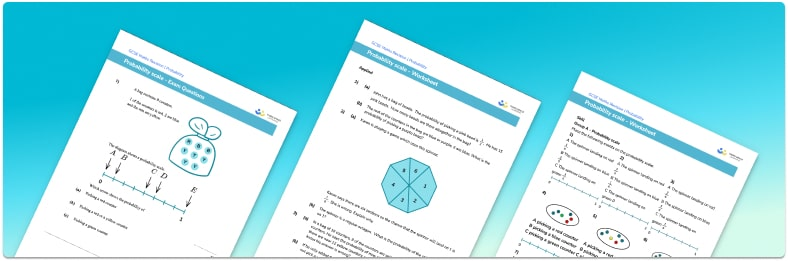# Probability Scale Worksheet• Section 1 of the probability scale worksheet contains 36 skills-based probability scale questions, in 3 groups to support differentiation
• Section 2 contains 3 applied probability scale questions with a mix of worded problems and deeper problem solving questions
• Section 3 contains 4 foundation and higher level GCSE exam style probability scale questions
• Answers and a mark scheme for all probability scale questions are provided
• Questions follow variation theory with plenty of opportunities for students to work independently at their own level
• All questions created by fully qualified expert secondary maths teachers
• Suitable for GCSE maths revision for AQA, OCR and Edexcel exam boards

• This field is for validation purposes and should be left unchanged.

You can unsubscribe at any time (each email we send will contain an easy way to unsubscribe). To find out more about how we use your data, see our privacy policy.

### Probability scale at a glance

The probability of an event occurring is how likely it is to happen. The probability words that we use are: certain, likely, even chance, unlikely and impossible. Probabilities are given on a scale from 0 to 1, with 0 being impossible, 1 being certain and 0.5 being an even chance. Probabilities can be written as fractions, decimals or percentages and we can indicate the probability of events by marking it on the probability scale (a number line).

An event is something that happens such as landing on a red sector of a fair spinner, picking a number that ends in a 5 or a 0 from a raffle draw, rolling a 5 on a fair dice, or picking a student who wears glasses. The probability of these events occurring would be expressed as a capital P followed by a set of round brackets in which the event lies inside the bracket, such as P(red), P(a number ending in 5 or 0), or P(glasses).

The location of an event on the probability scale is marked at the point describing how likely the event is to occur. More likely outcomes will be closer to 1 on the probability scale; less likely outcomes would be closer to 0.

To determine the exact probability of an event occurring, we divide the number of required outcomes by the total number of outcomes. For example, if we want to find the probability that a fair dice lands on a prime number, there are 3 prime numbers on the dice (2, 3, and 5) and 6 numbers altogether. Therefore the probability the dice lands on a prime number is 3 out of 6, or one half. The probability of rolling an odd number is one half; the probability of rolling an even number is also one half.

To find the probability of an event not occurring, we subtract the probability that they do occur from 1. For example, a bag contains 3 blue counters, 4 green counters and 5 red counters. The probability of picking a blue counter from the bag is 3 out of 12. The probability of not picking a blue counter is found by subtracting 3 out of 12 from 1 to get 9 out of 12. This is the same as calculating the probability of picking a red or green counter from the bag.

If events are exhaustive events then they cover all possible options. For example, rolling an even number and rolling an odd number on a dice are exhaustive events since any number we roll will either be even or odd, there are no other possibilities.

Looking forward, students can then progress to additional probability worksheets, for example the Venn diagram worksheet, the probability tree diagram worksheet or the conditional probability worksheet.For more teaching and learning support on Probability our GCSE maths lessons provide step by step support for all GCSE maths concepts.

## Do you have KS4 students who need more focused attention to succeed at GCSE?There will be students in your class who require individual attention to help them succeed in their maths GCSEs. In a class of 30, it’s not always easy to provide.

Help your students feel confident with exam-style questions and the strategies they’ll need to answer them correctly with our dedicated GCSE maths revision programme.

Lessons are selected to provide support where each student needs it most, and specially-trained GCSE maths tutors adapt the pitch and pace of each lesson. This ensures a personalised revision programme that raises grades and boosts confidence.

Find out more